# RD Sharma Solutions for Class 11 Maths Chapter 27 Hyperbola

## RD Sharma Solutions Class 11 Maths Chapter 27 – Download Free PDF Updated for (2021-22)

RD Sharma Solutions for Class 11 Maths Chapter 27 – Hyperbola are provided here for students to score good marks in the board exams. We have discussed in earlier chapters that a hyperbola is a particular case of conic. Now in this section, we shall study about hyperbola in detail by finding the equation of the Hyperbola in standard form. Exercise-wise solutions are provided to help students understand the concepts clearly from the exam point of view.

Experts have designed RD Sharma Solutions where the concepts are explained in detail, which is very helpful in preparing for their board exams. Students who find difficulty in solving problems can quickly jump to RD Sharma Class 11 Maths Solutions, download the pdf from the links given below and can start practising offline for good results.

Chapter 27 – Hyperbola contains one exercise and the RD Sharma Solutions present in this page provide solutions to the questions present in this exercise. Now, let us have a look at the concepts discussed in this chapter.

• Equation of the hyperbola in standard form.
• Tracing of a hyperbola.
• Second focus and second directrix of the hyperbola.
• Various elements of a hyperbola.
• Eccentricity.
• Length of the latus-rectum.
• Focal distances of a point.
• Conjugate hyperbola.

## Download the pdf of RD Sharma Solutions for Class 11 Maths Chapter 27 – Hyperbola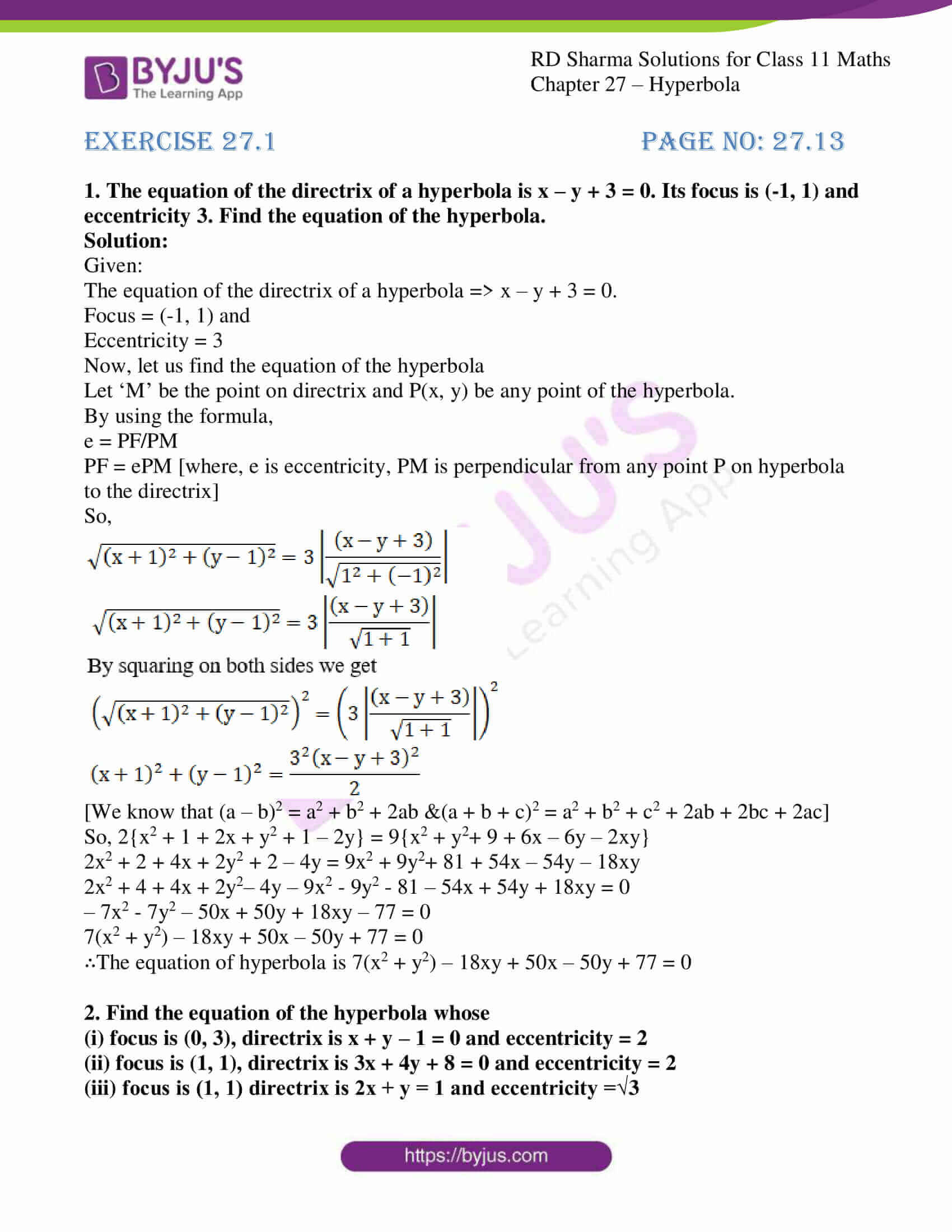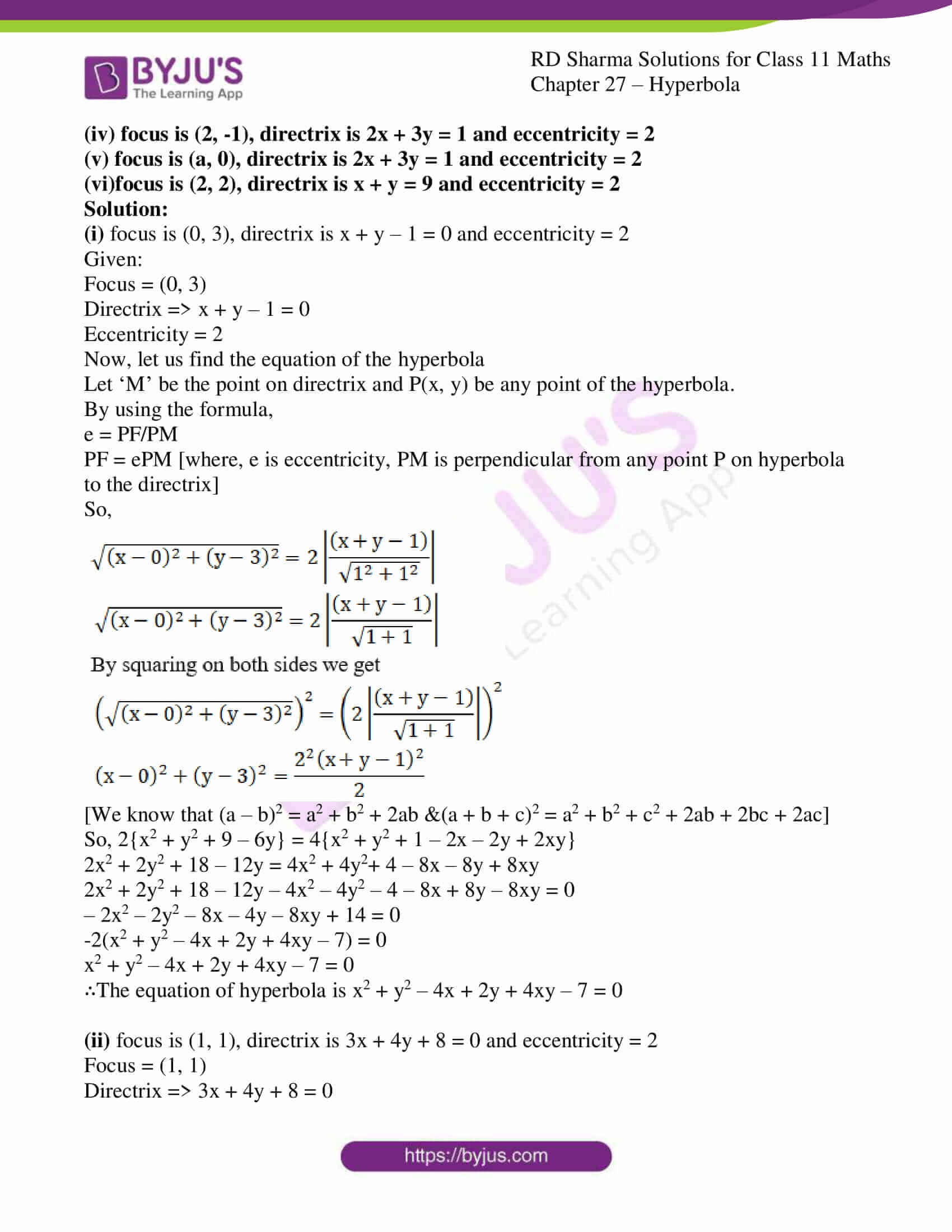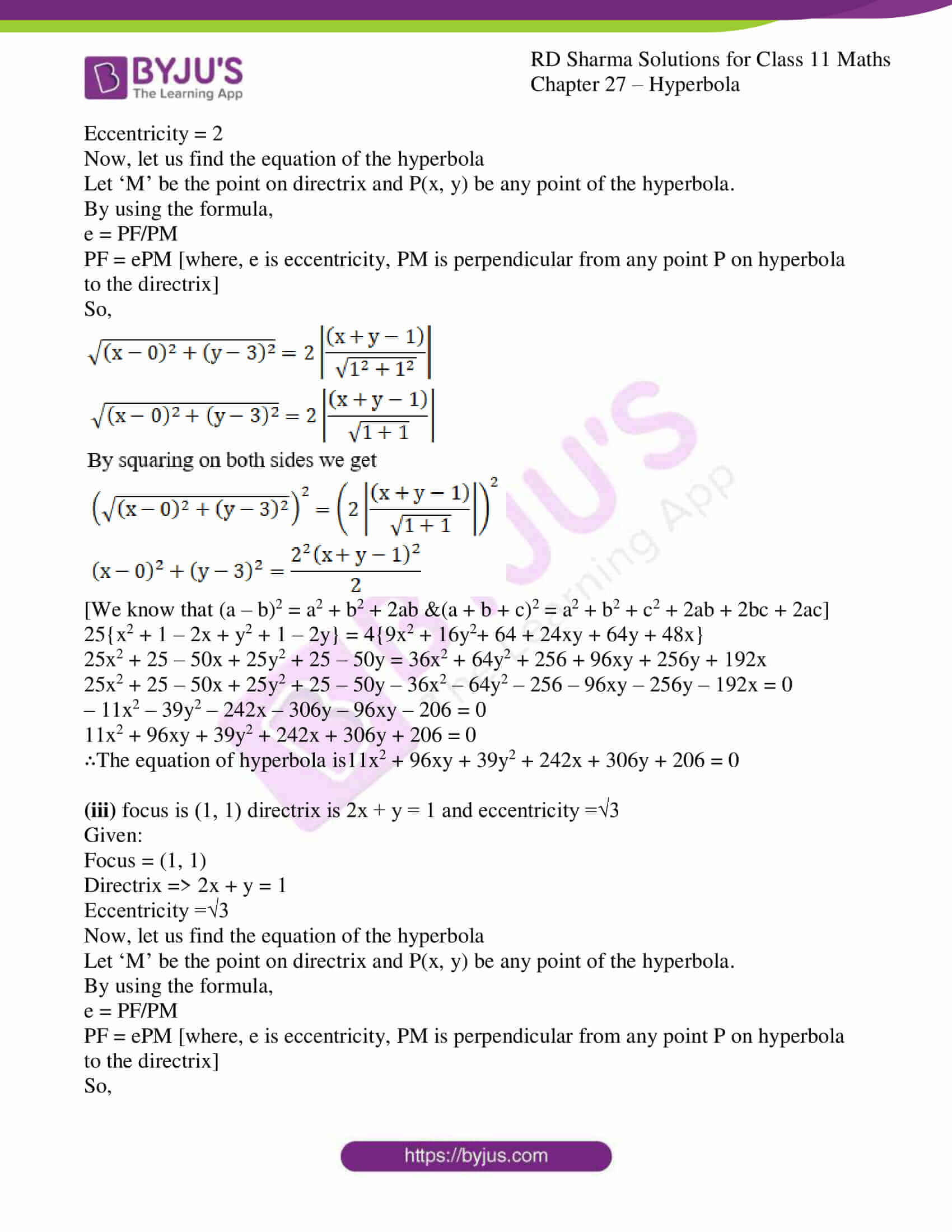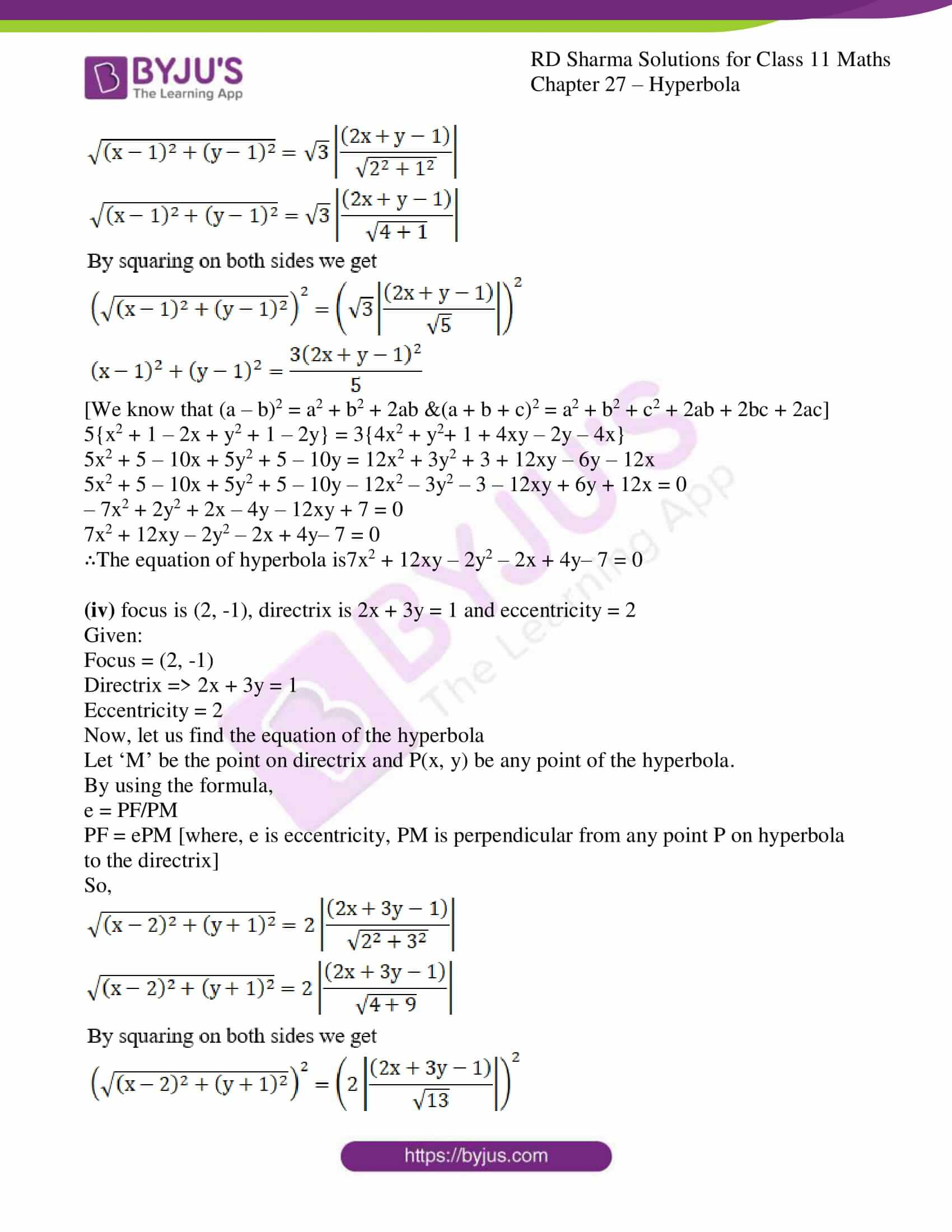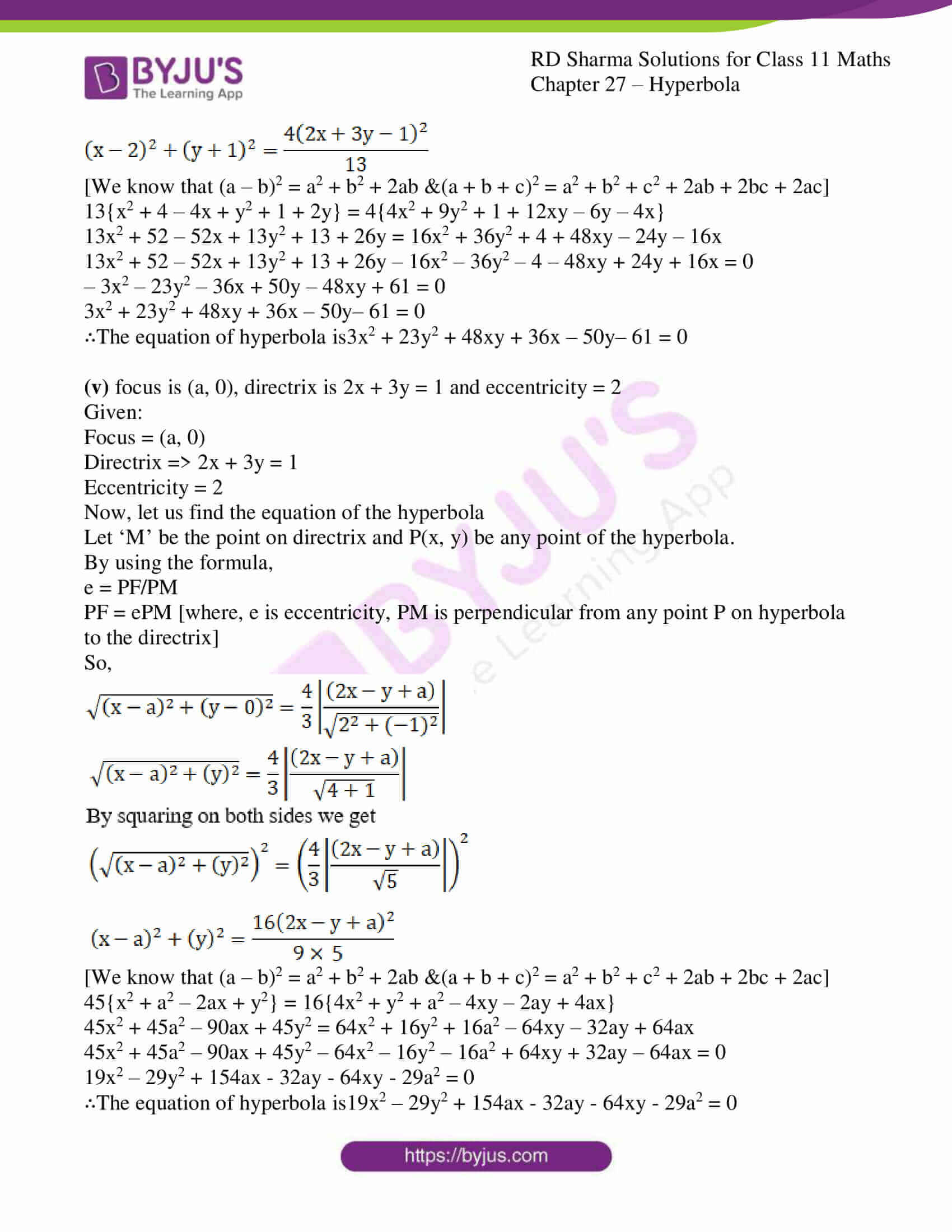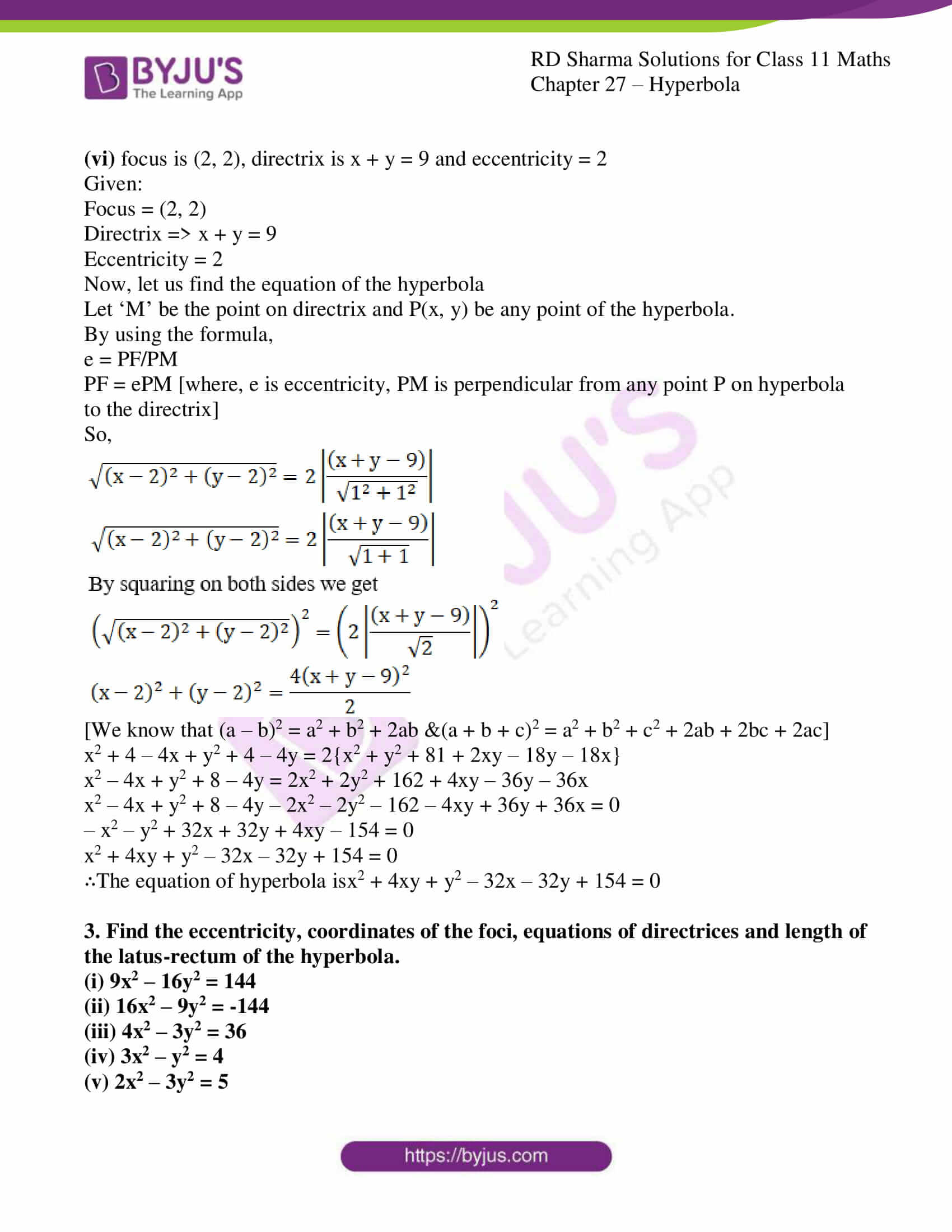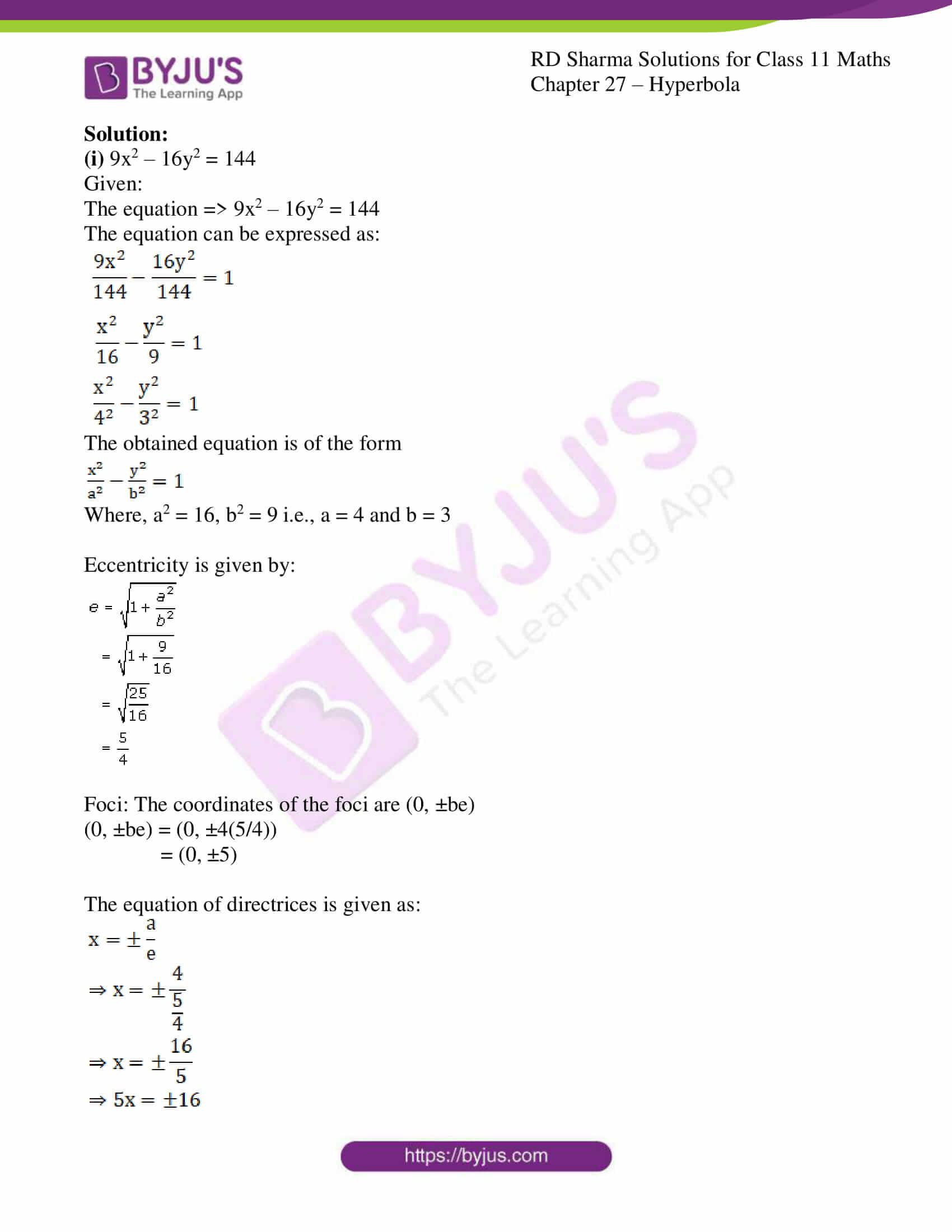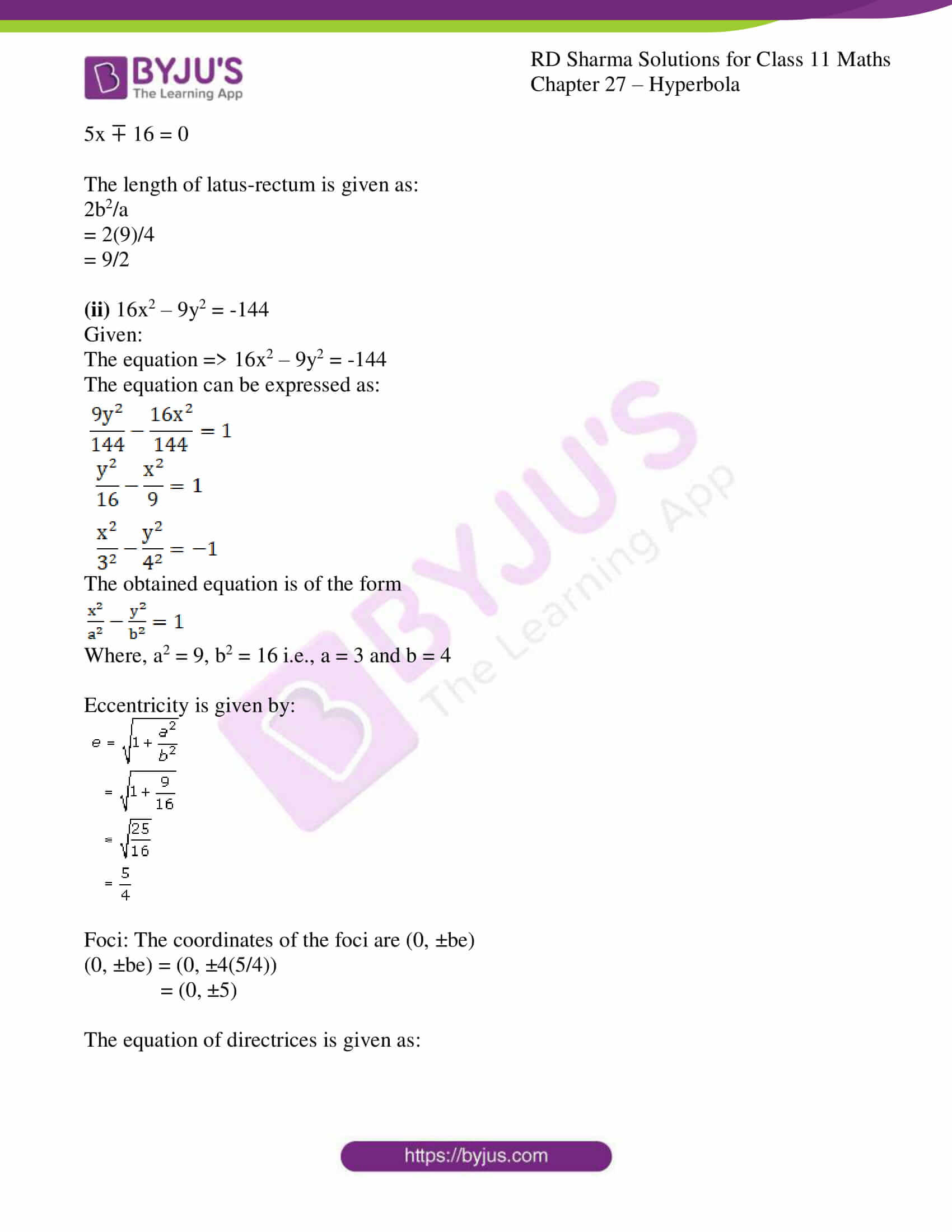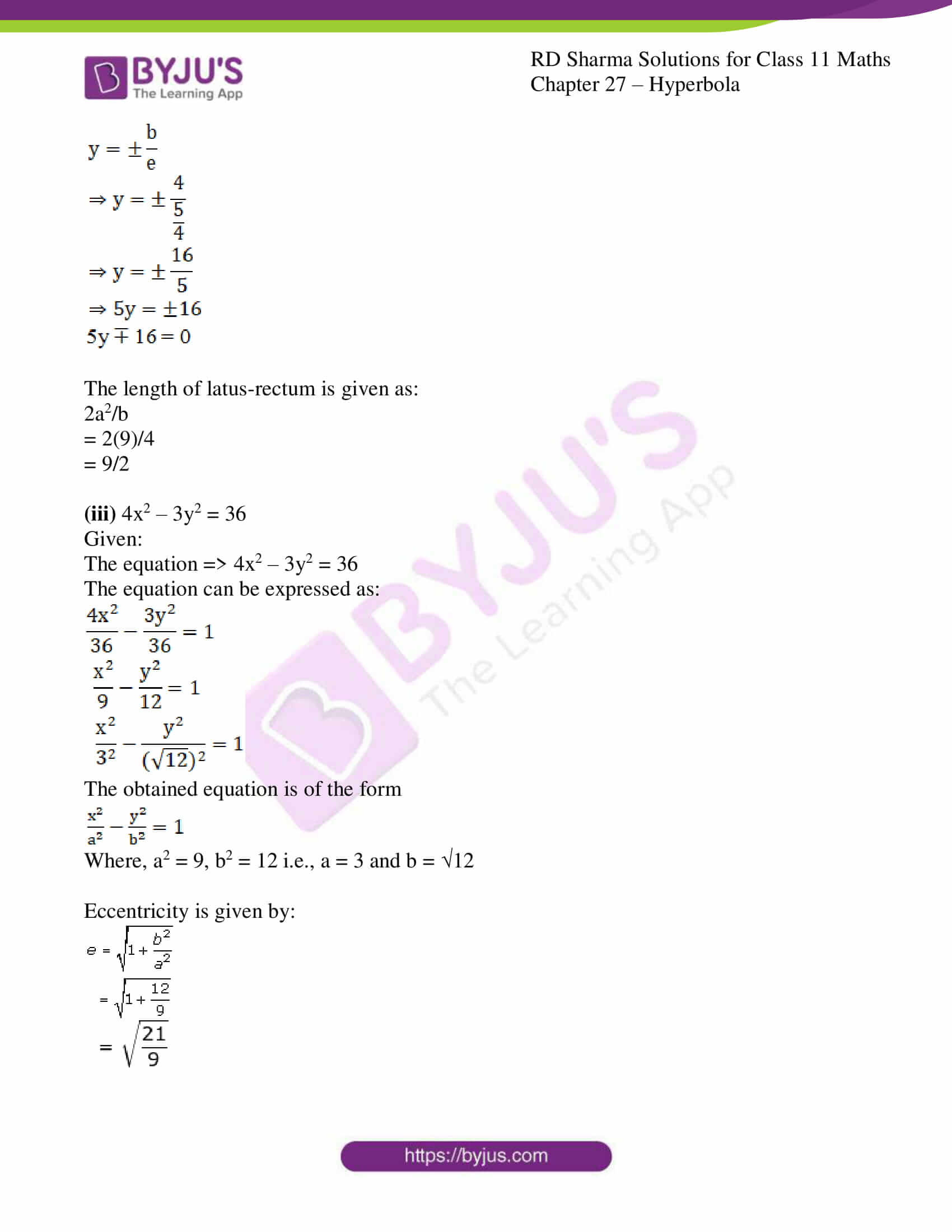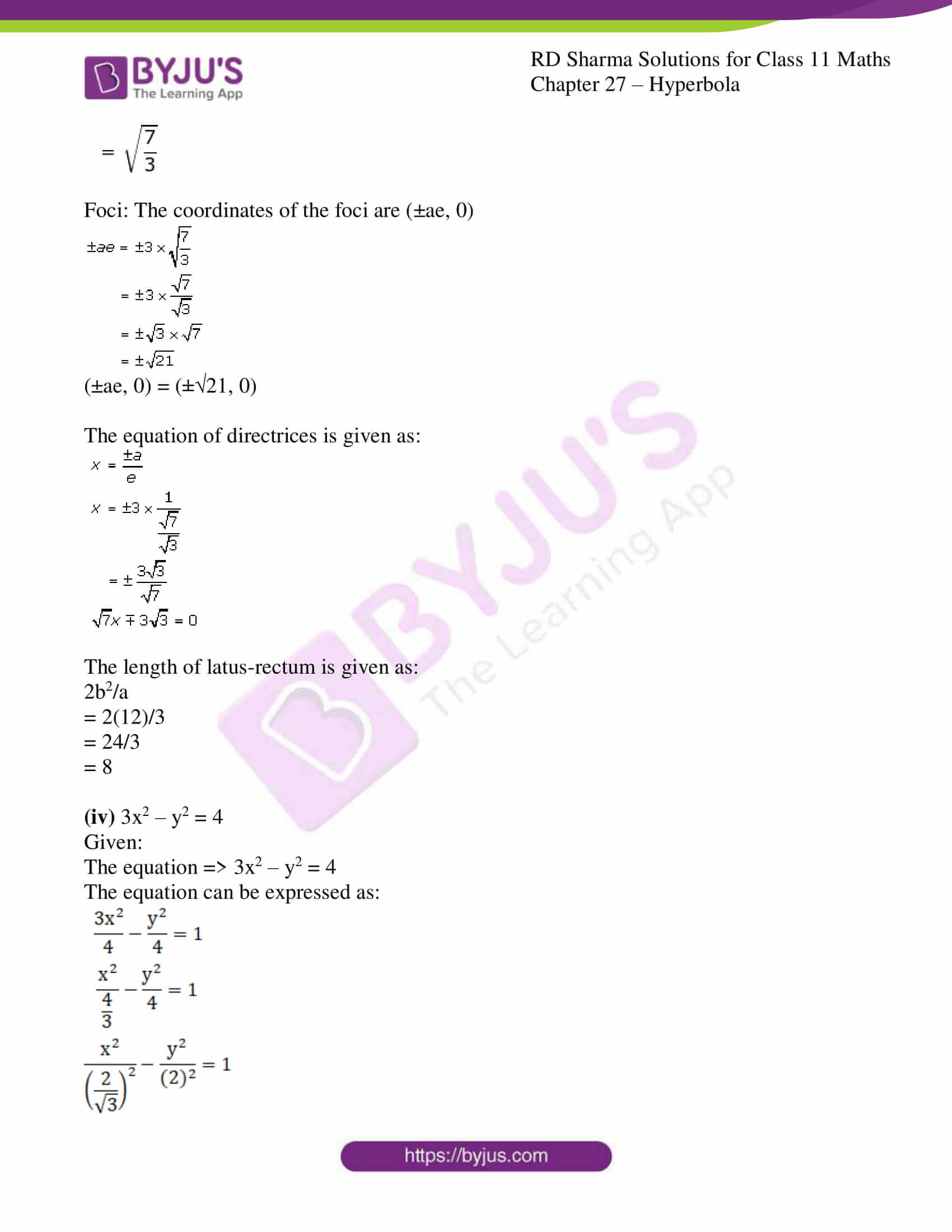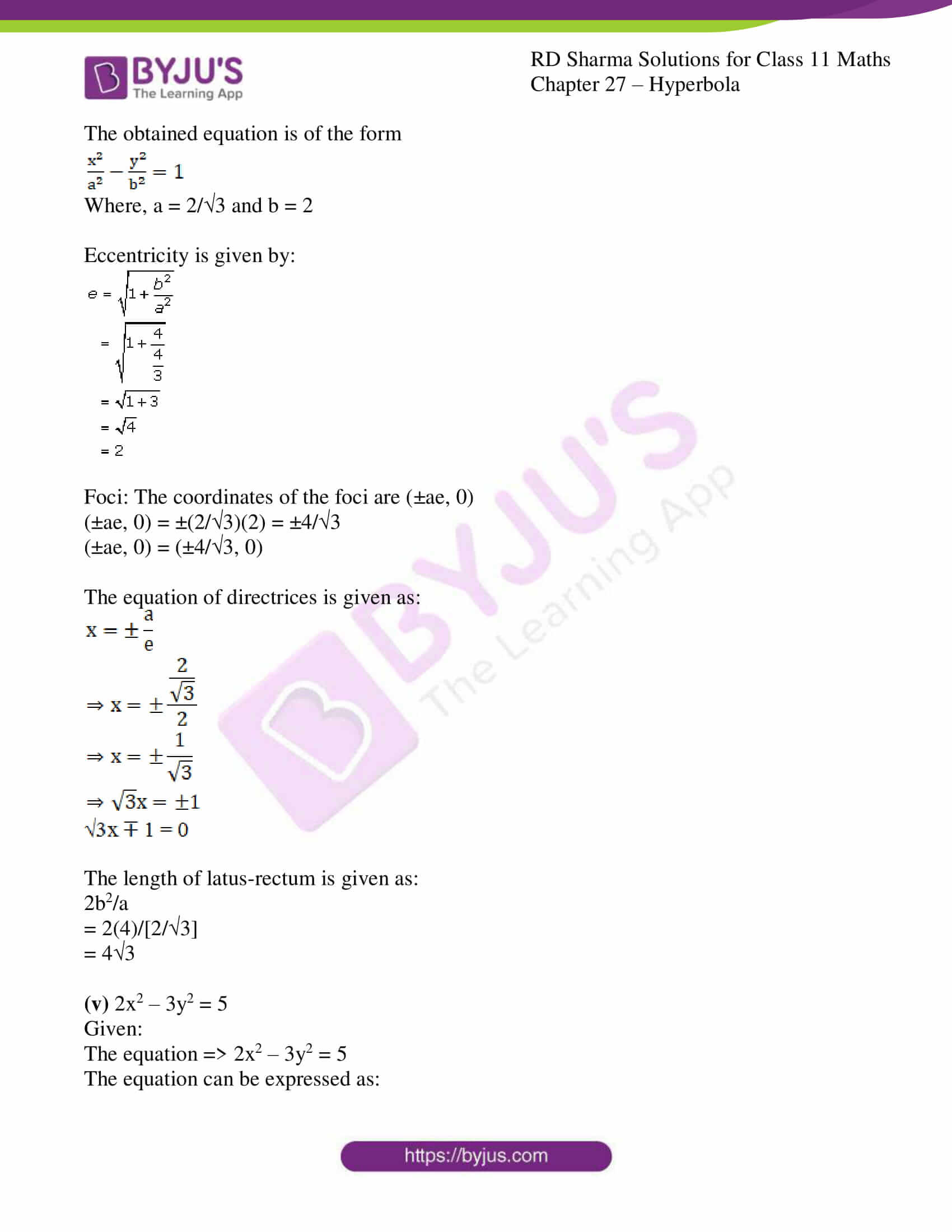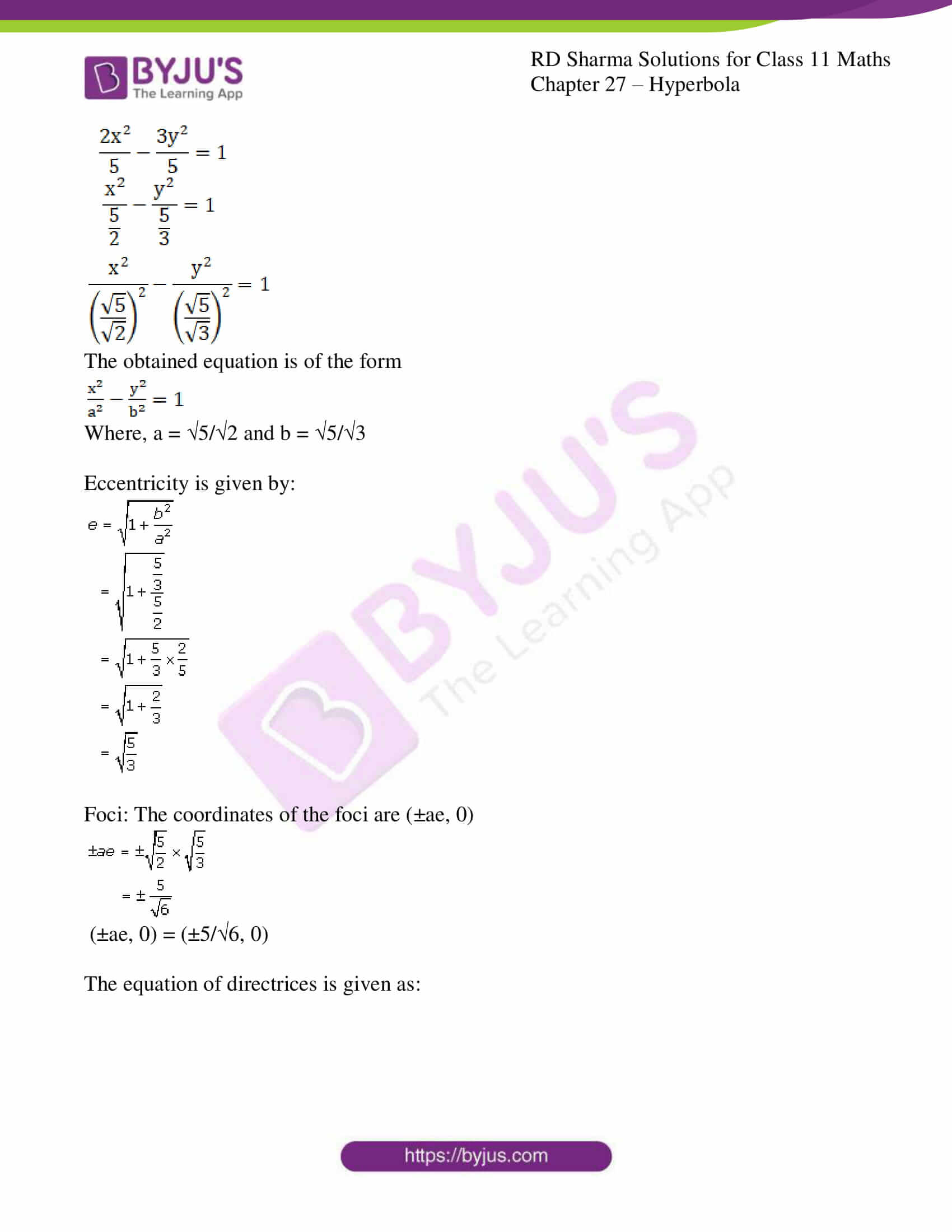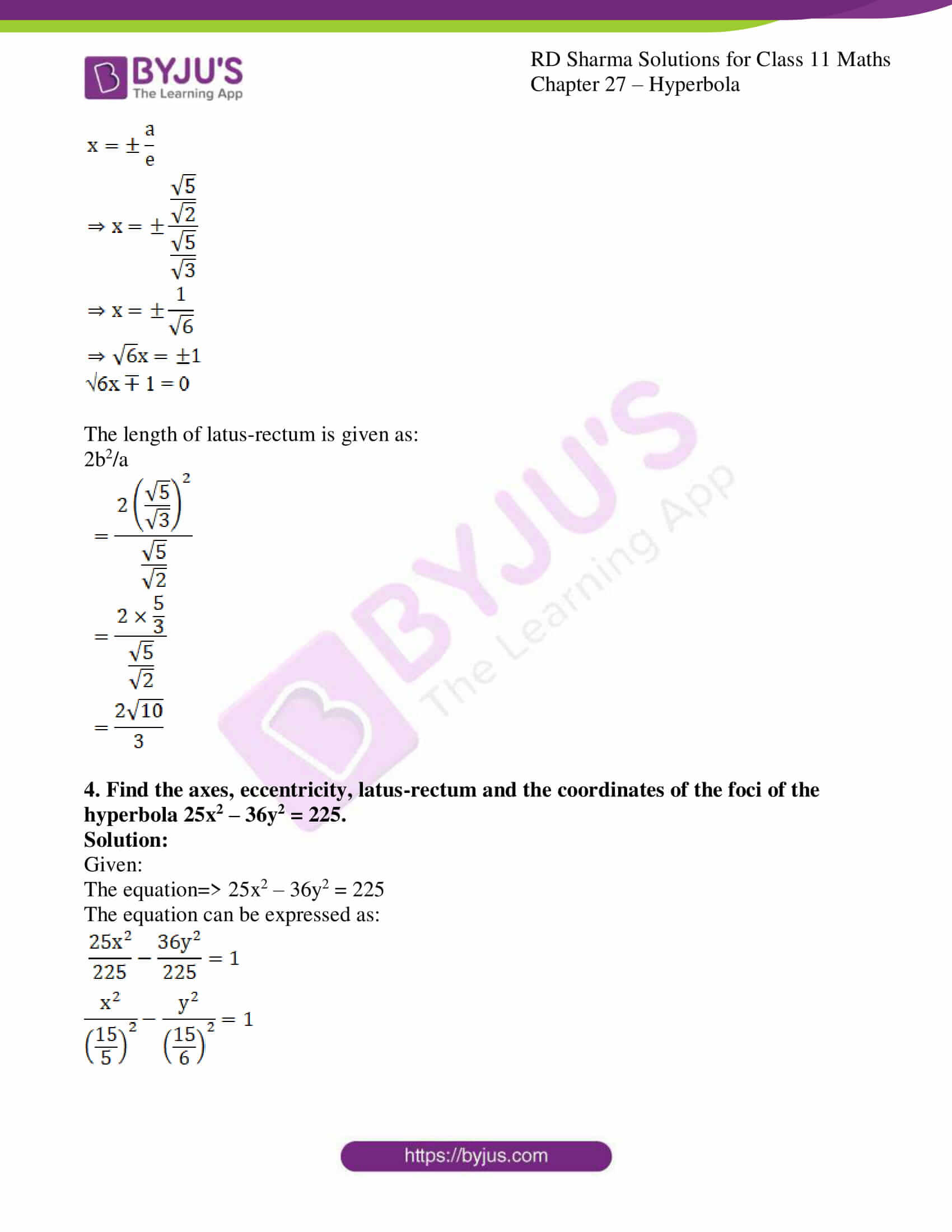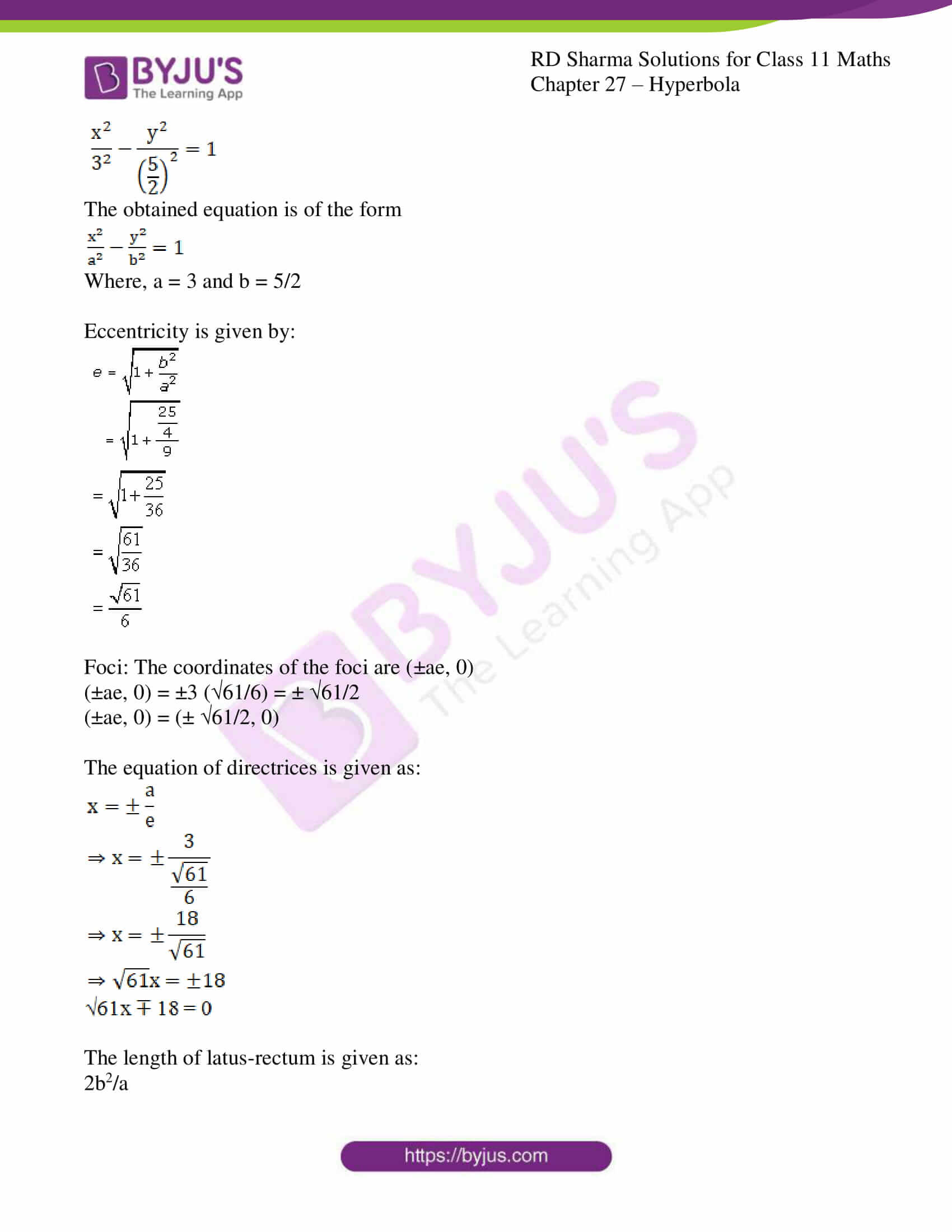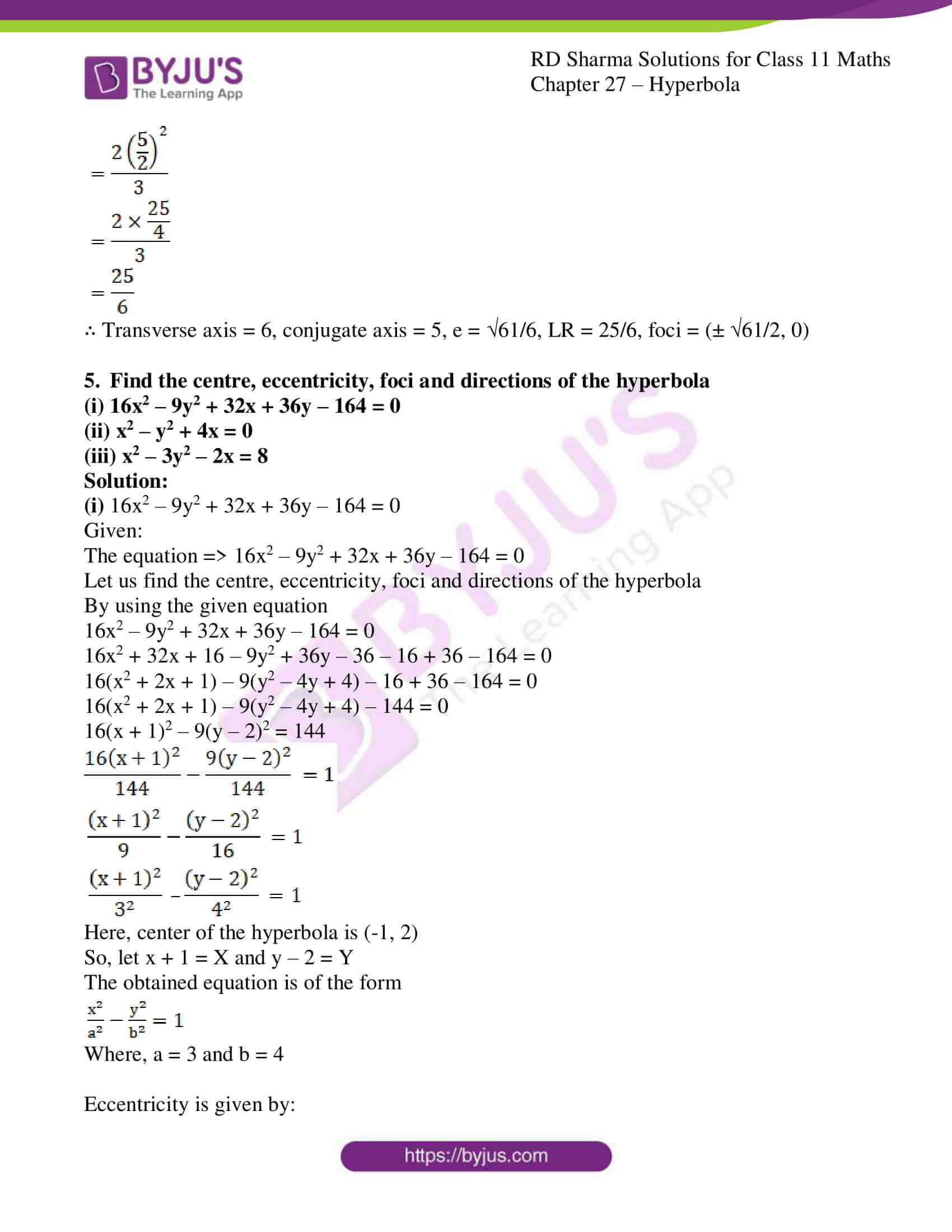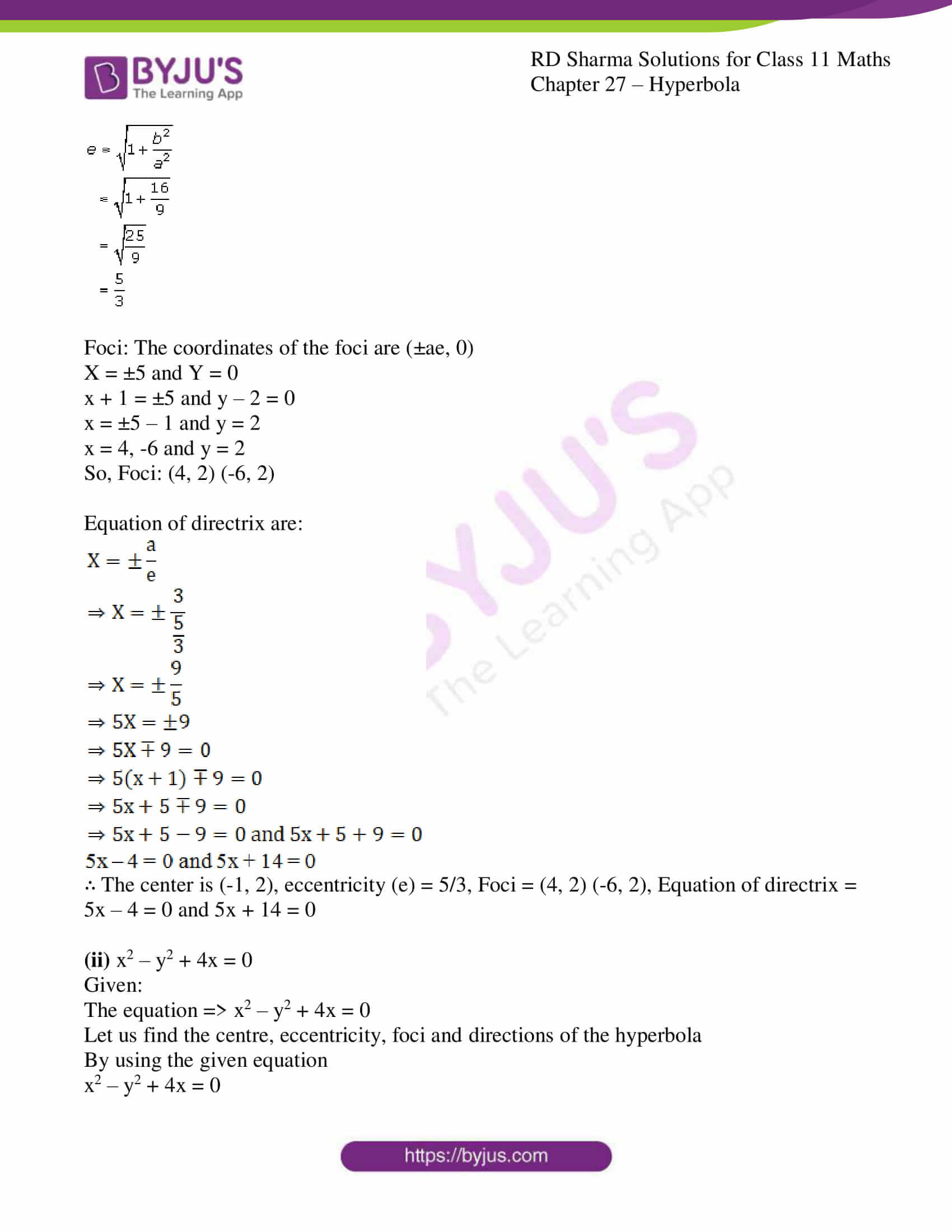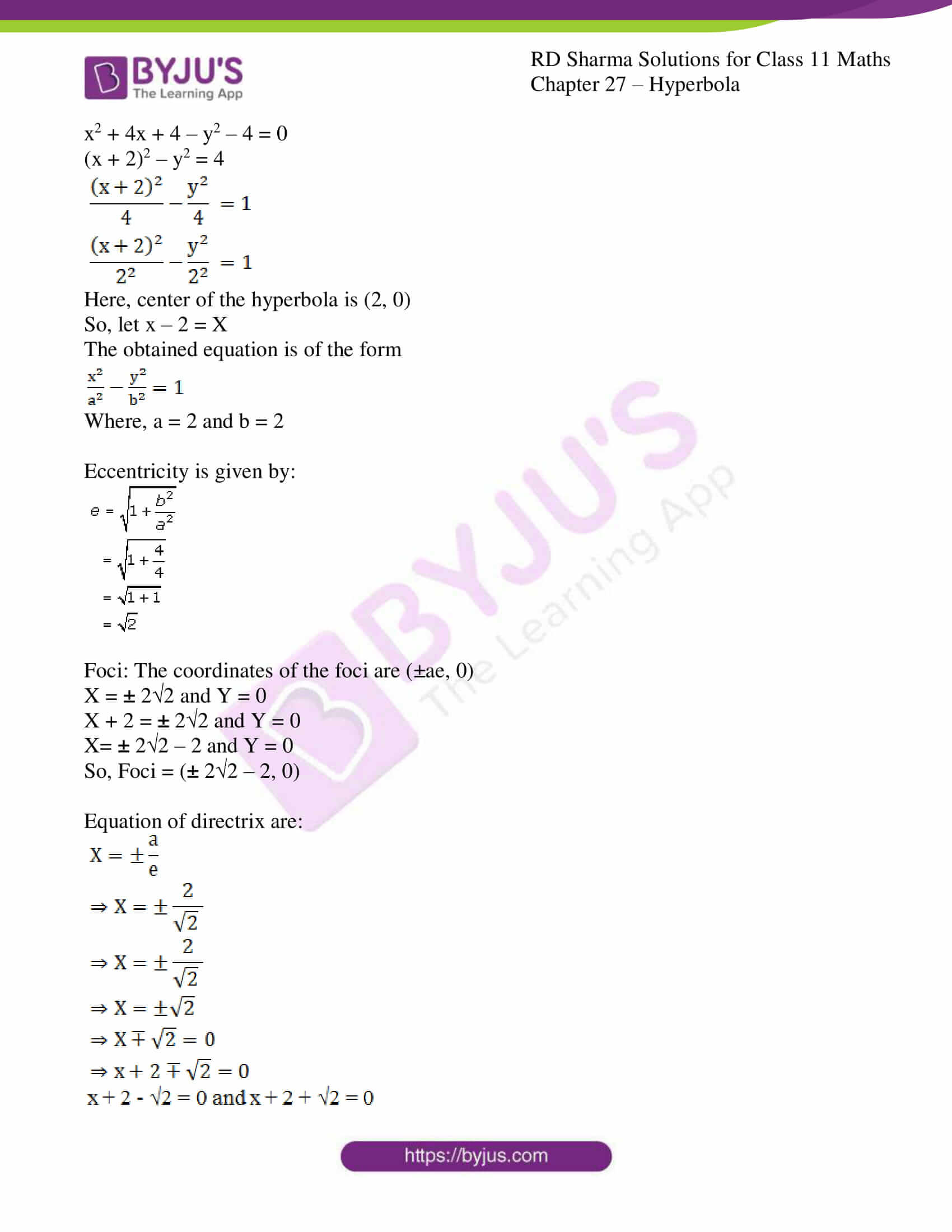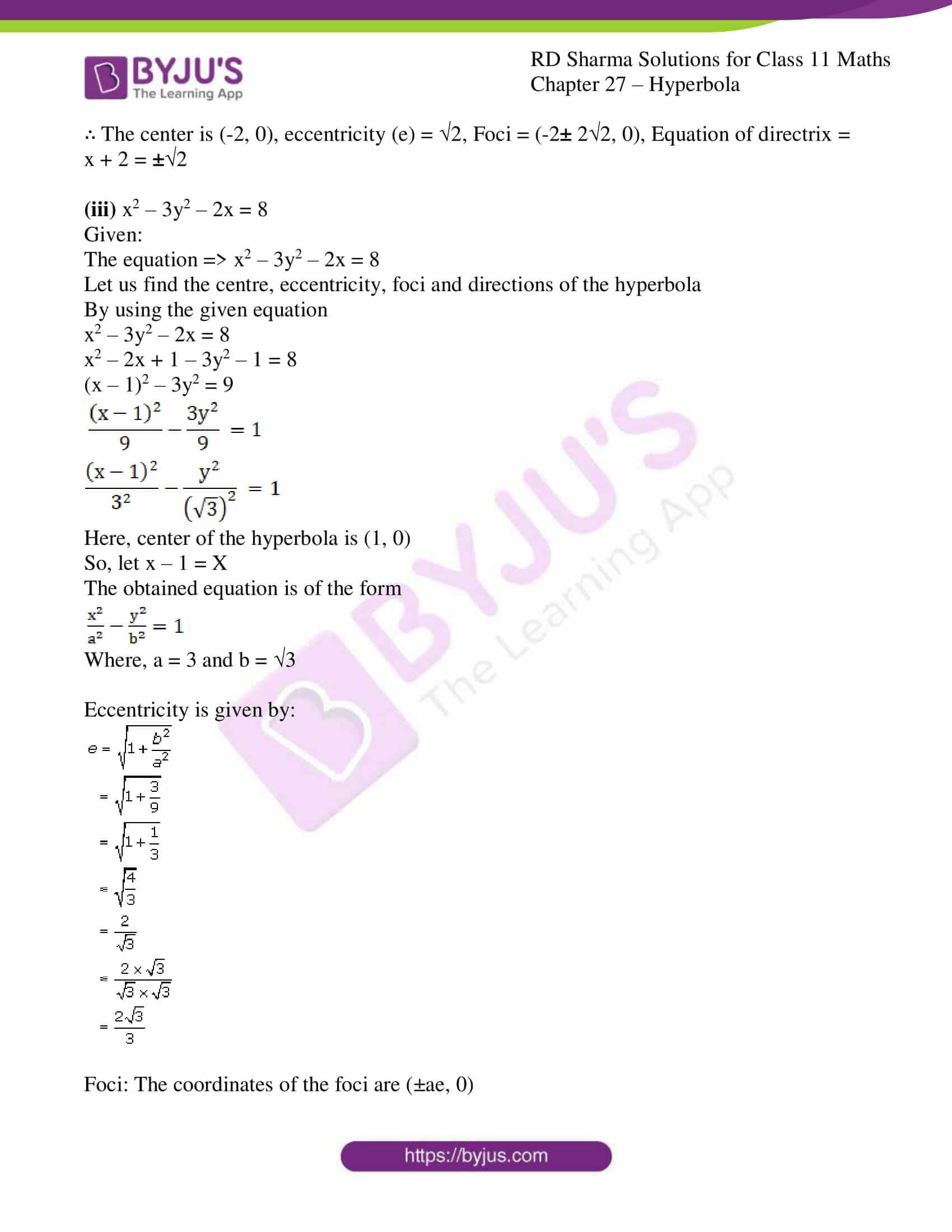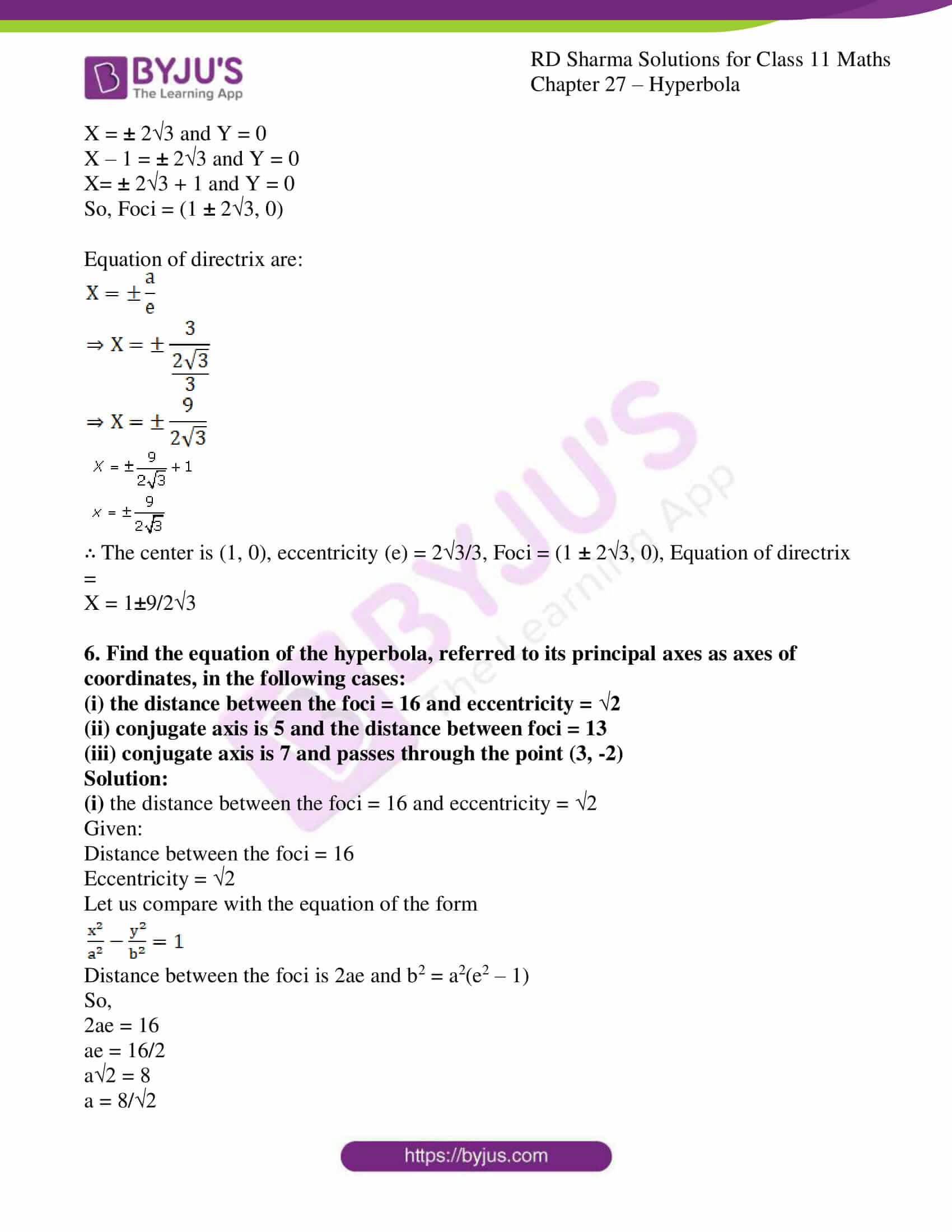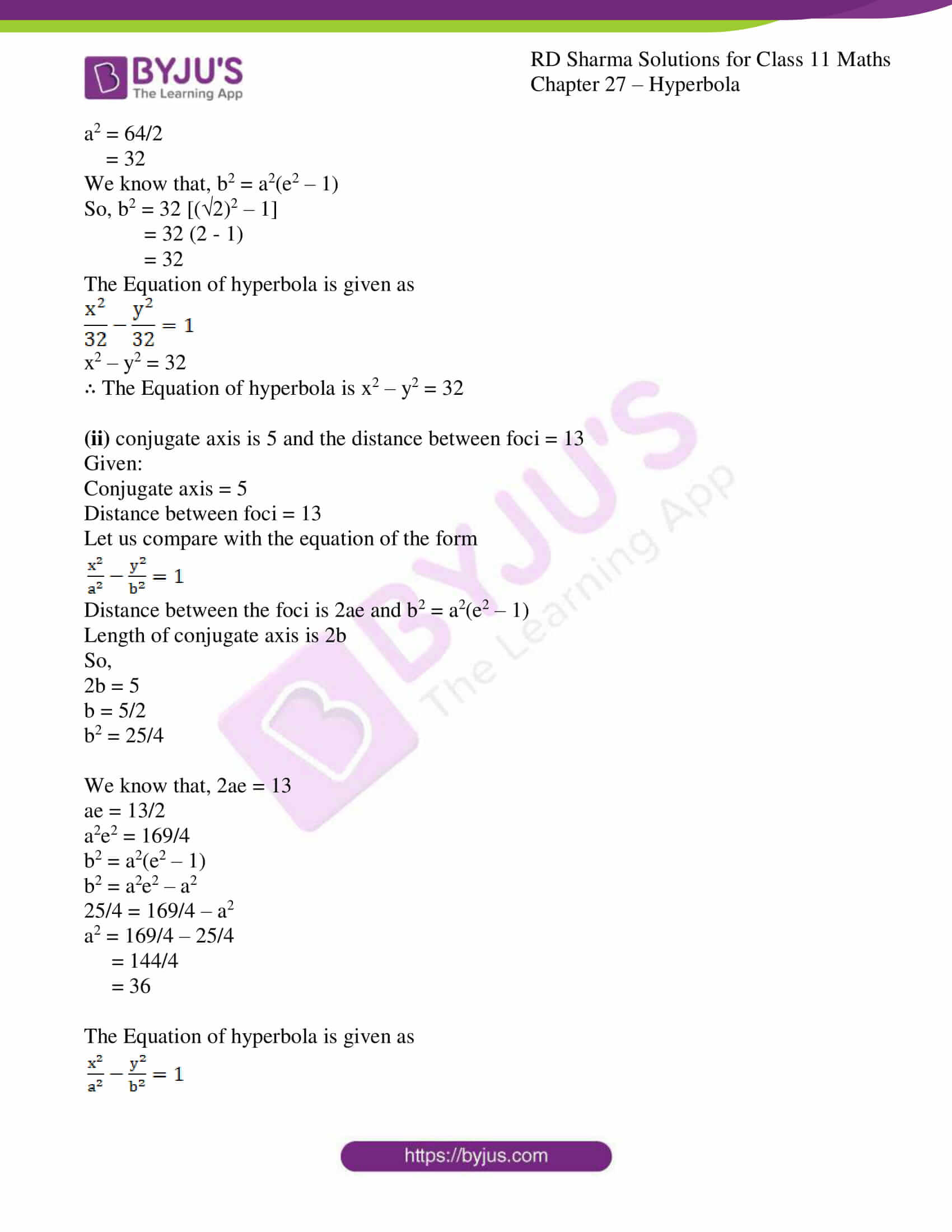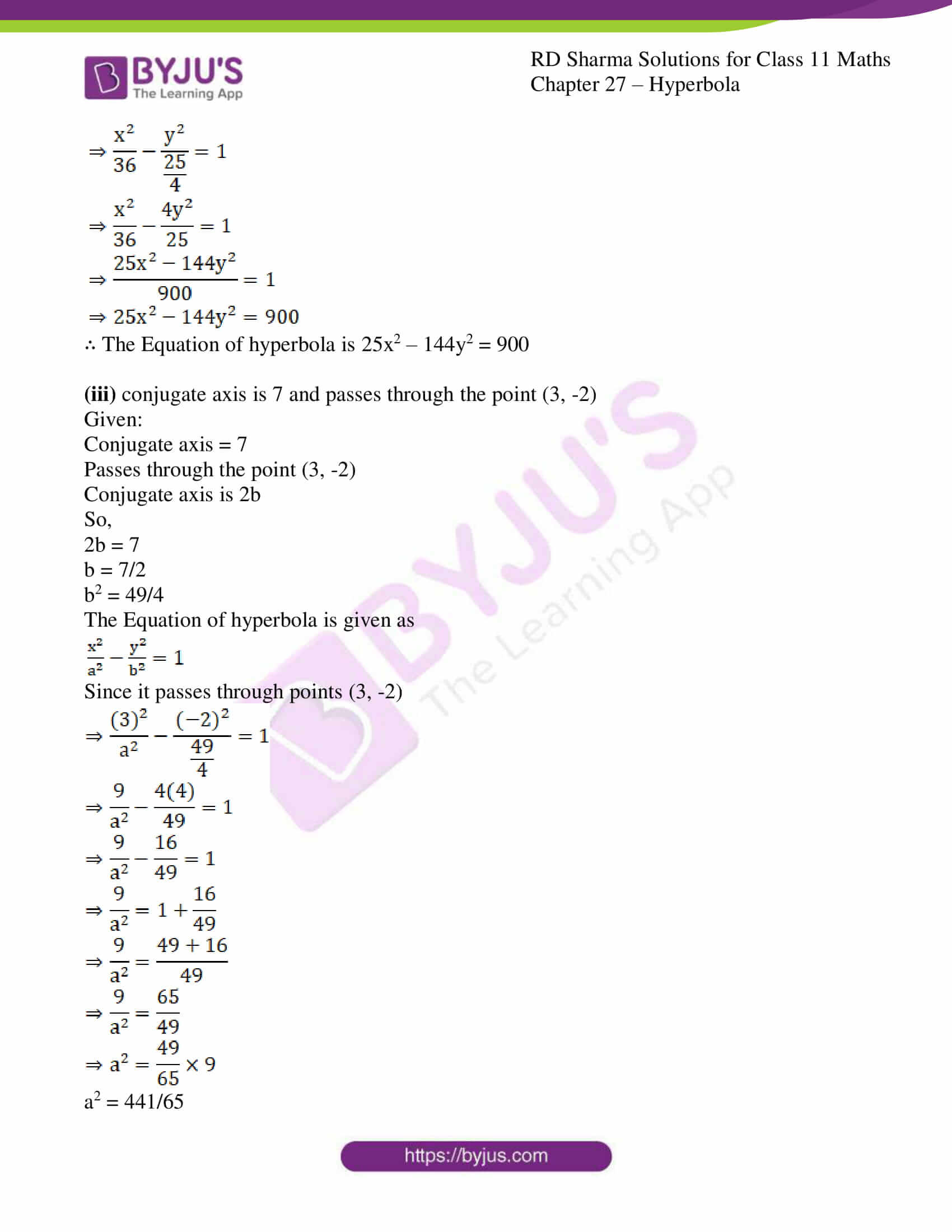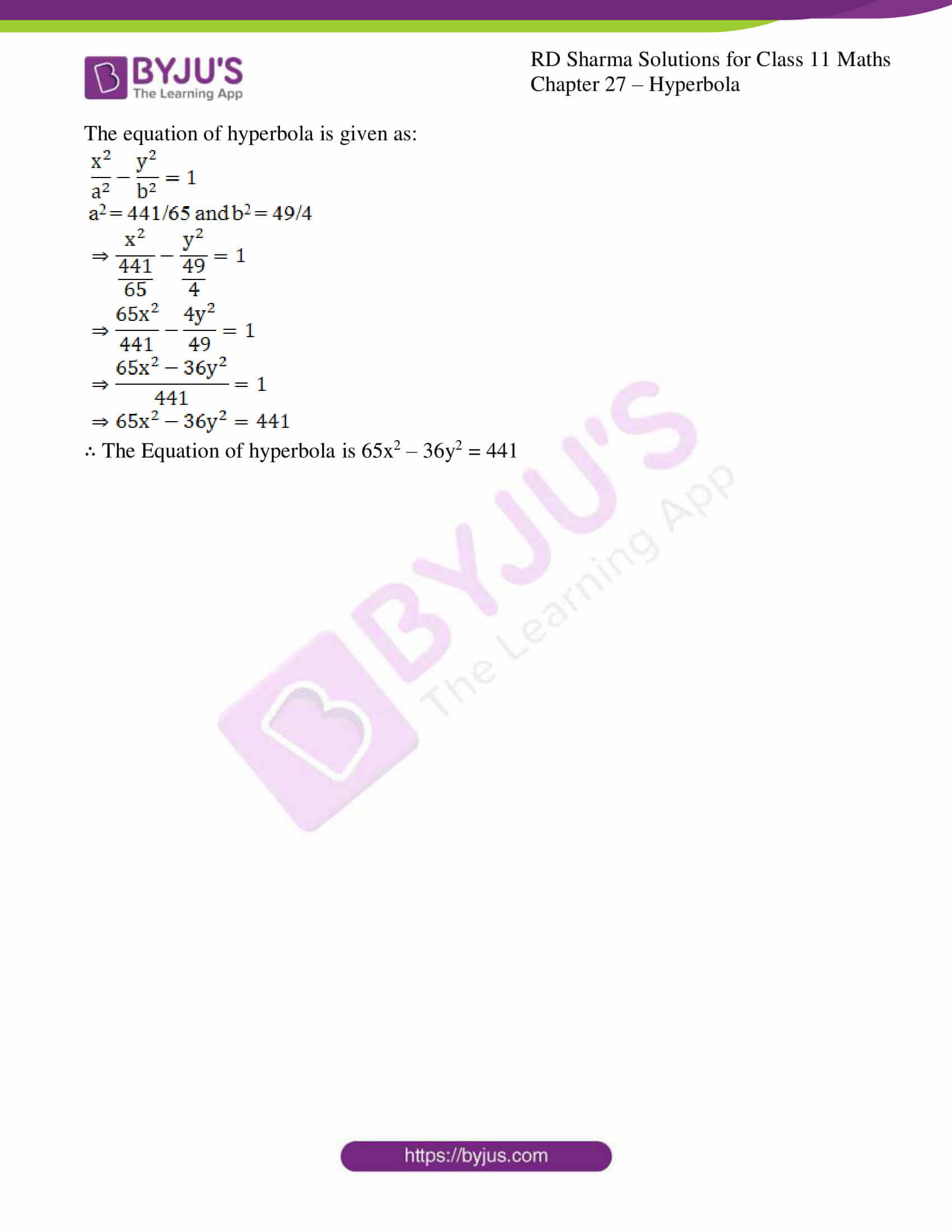### Access RD Sharma Solutions for Class 11 Maths Chapter 27 – Hyperbola

EXERCISE 27.1 PAGE NO: 27.13

1. The equation of the directrix of a hyperbola is x – y + 3 = 0. Its focus is (-1, 1) and eccentricity 3. Find the equation of the hyperbola.

Solution:

Given:

The equation of the directrix of a hyperbola => x – y + 3 = 0.

Focus = (-1, 1) and

Eccentricity = 3

Now, let us find the equation of the hyperbola

Let ‘M’ be the point on directrix and P(x, y) be any point of the hyperbola.

By using the formula,

e = PF/PM

PF = ePM [where, e is eccentricity, PM is perpendicular from any point P on hyperbola to the directrix]

So,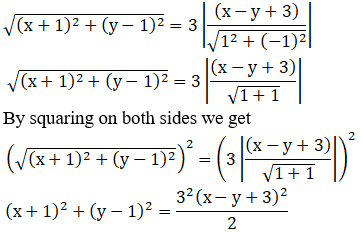[We know that (a – b)2 = a2 + b2 + 2ab &(a + b + c)2 = a2 + b2 + c2 + 2ab + 2bc + 2ac]

So, 2{x2 + 1 + 2x + y2 + 1 – 2y} = 9{x2 + y2+ 9 + 6x – 6y – 2xy}

2x2 + 2 + 4x + 2y2 + 2 – 4y = 9x2 + 9y2+ 81 + 54x – 54y – 18xy

2x2 + 4 + 4x + 2y2– 4y – 9x2 – 9y2 – 81 – 54x + 54y + 18xy = 0

– 7x2 – 7y2 – 50x + 50y + 18xy – 77 = 0

7(x2 + y2) – 18xy + 50x – 50y + 77 = 0

∴The equation of hyperbola is 7(x2 + y2) – 18xy + 50x – 50y + 77 = 0

2. Find the equation of the hyperbola whose

(i) focus is (0, 3), directrix is x + y – 1 = 0 and eccentricity = 2

(ii) focus is (1, 1), directrix is 3x + 4y + 8 = 0 and eccentricity = 2

(iii) focus is (1, 1) directrix is 2x + y = 1 and eccentricity =√3

(iv) focus is (2, -1), directrix is 2x + 3y = 1 and eccentricity = 2

(v) focus is (a, 0), directrix is 2x + 3y = 1 and eccentricity = 2

(vi)focus is (2, 2), directrix is x + y = 9 and eccentricity = 2

Solution:

(i) focus is (0, 3), directrix is x + y – 1 = 0 and eccentricity = 2

Given:

Focus = (0, 3)

Directrix => x + y – 1 = 0

Eccentricity = 2

Now, let us find the equation of the hyperbola

Let ‘M’ be the point on directrix and P(x, y) be any point of the hyperbola.

By using the formula,

e = PF/PM

PF = ePM [where, e is eccentricity, PM is perpendicular from any point P on hyperbola to the directrix]

So,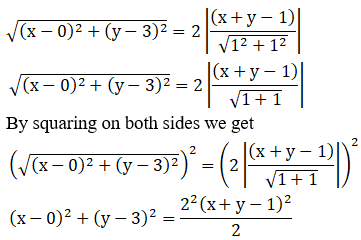[We know that (a – b)2 = a2 + b2 + 2ab &(a + b + c)2 = a2 + b2 + c2 + 2ab + 2bc + 2ac]

So, 2{x2 + y2 + 9 – 6y} = 4{x2 + y2 + 1 – 2x – 2y + 2xy}

2x2 + 2y2 + 18 – 12y = 4x2 + 4y2+ 4 – 8x – 8y + 8xy

2x2 + 2y2 + 18 – 12y – 4x2 – 4y2 – 4 – 8x + 8y – 8xy = 0

– 2x2 – 2y2 – 8x – 4y – 8xy + 14 = 0

-2(x2 + y2 – 4x + 2y + 4xy – 7) = 0

x2 + y2 – 4x + 2y + 4xy – 7 = 0

∴The equation of hyperbola is x2 + y2 – 4x + 2y + 4xy – 7 = 0

(ii) focus is (1, 1), directrix is 3x + 4y + 8 = 0 and eccentricity = 2

Focus = (1, 1)

Directrix => 3x + 4y + 8 = 0

Eccentricity = 2

Now, let us find the equation of the hyperbola

Let ‘M’ be the point on directrix and P(x, y) be any point of the hyperbola.

By using the formula,

e = PF/PM

PF = ePM [where, e is eccentricity, PM is perpendicular from any point P on hyperbola to the directrix]

So,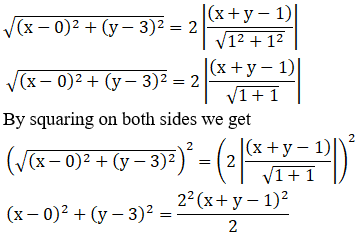[We know that (a – b)2 = a2 + b2 + 2ab &(a + b + c)2 = a2 + b2 + c2 + 2ab + 2bc + 2ac]

25{x2 + 1 – 2x + y2 + 1 – 2y} = 4{9x2 + 16y2+ 64 + 24xy + 64y + 48x}

25x2 + 25 – 50x + 25y2 + 25 – 50y = 36x2 + 64y2 + 256 + 96xy + 256y + 192x

25x2 + 25 – 50x + 25y2 + 25 – 50y – 36x2 – 64y2 – 256 – 96xy – 256y – 192x = 0

– 11x2 – 39y2 – 242x – 306y – 96xy – 206 = 0

11x2 + 96xy + 39y2 + 242x + 306y + 206 = 0

∴The equation of hyperbola is11x2 + 96xy + 39y2 + 242x + 306y + 206 = 0

(iii) focus is (1, 1) directrix is 2x + y = 1 and eccentricity =√3

Given:

Focus = (1, 1)

Directrix => 2x + y = 1

Eccentricity =√3

Now, let us find the equation of the hyperbola

Let ‘M’ be the point on directrix and P(x, y) be any point of the hyperbola.

By using the formula,

e = PF/PM

PF = ePM [where, e is eccentricity, PM is perpendicular from any point P on hyperbola to the directrix]

So,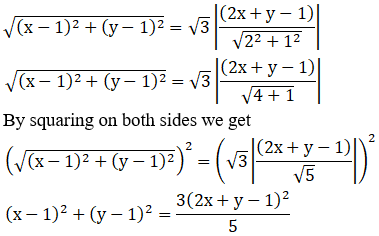[We know that (a – b)2 = a2 + b2 + 2ab &(a + b + c)2 = a2 + b2 + c2 + 2ab + 2bc + 2ac]

5{x2 + 1 – 2x + y2 + 1 – 2y} = 3{4x2 + y2+ 1 + 4xy – 2y – 4x}

5x2 + 5 – 10x + 5y2 + 5 – 10y = 12x2 + 3y2 + 3 + 12xy – 6y – 12x

5x2 + 5 – 10x + 5y2 + 5 – 10y – 12x2 – 3y2 – 3 – 12xy + 6y + 12x = 0

– 7x2 + 2y2 + 2x – 4y – 12xy + 7 = 0

7x2 + 12xy – 2y2 – 2x + 4y– 7 = 0

∴The equation of hyperbola is7x2 + 12xy – 2y2 – 2x + 4y– 7 = 0

(iv) focus is (2, -1), directrix is 2x + 3y = 1 and eccentricity = 2

Given:

Focus = (2, -1)

Directrix => 2x + 3y = 1

Eccentricity = 2

Now, let us find the equation of the hyperbola

Let ‘M’ be the point on directrix and P(x, y) be any point of the hyperbola.

By using the formula,

e = PF/PM

PF = ePM [where, e is eccentricity, PM is perpendicular from any point P on hyperbola to the directrix]

So,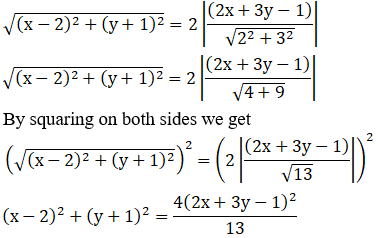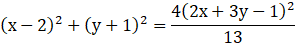[We know that (a – b)2 = a2 + b2 + 2ab &(a + b + c)2 = a2 + b2 + c2 + 2ab + 2bc + 2ac]

13{x2 + 4 – 4x + y2 + 1 + 2y} = 4{4x2 + 9y2 + 1 + 12xy – 6y – 4x}

13x2 + 52 – 52x + 13y2 + 13 + 26y = 16x2 + 36y2 + 4 + 48xy – 24y – 16x

13x2 + 52 – 52x + 13y2 + 13 + 26y – 16x2 – 36y2 – 4 – 48xy + 24y + 16x = 0

– 3x2 – 23y2 – 36x + 50y – 48xy + 61 = 0

3x2 + 23y2 + 48xy + 36x – 50y– 61 = 0

∴The equation of hyperbola is3x2 + 23y2 + 48xy + 36x – 50y– 61 = 0

(v) focus is (a, 0), directrix is 2x + 3y = 1 and eccentricity = 2

Given:

Focus = (a, 0)

Directrix => 2x + 3y = 1

Eccentricity = 2

Now, let us find the equation of the hyperbola

Let ‘M’ be the point on directrix and P(x, y) be any point of the hyperbola.

By using the formula,

e = PF/PM

PF = ePM [where, e is eccentricity, PM is perpendicular from any point P on hyperbola to the directrix]

So,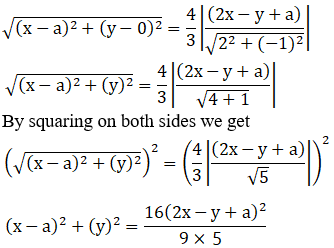[We know that (a – b)2 = a2 + b2 + 2ab &(a + b + c)2 = a2 + b2 + c2 + 2ab + 2bc + 2ac]

45{x2 + a2 – 2ax + y2} = 16{4x2 + y2 + a2 – 4xy – 2ay + 4ax}

45x2 + 45a2 – 90ax + 45y2 = 64x2 + 16y2 + 16a2 – 64xy – 32ay + 64ax

45x2 + 45a2 – 90ax + 45y2 – 64x2 – 16y2 – 16a2 + 64xy + 32ay – 64ax = 0

19x2 – 29y2 + 154ax – 32ay – 64xy – 29a2 = 0

∴The equation of hyperbola is19x2 – 29y2 + 154ax – 32ay – 64xy – 29a2 = 0

(vi) focus is (2, 2), directrix is x + y = 9 and eccentricity = 2

Given:

Focus = (2, 2)

Directrix => x + y = 9

Eccentricity = 2

Now, let us find the equation of the hyperbola

Let ‘M’ be the point on directrix and P(x, y) be any point of the hyperbola.

By using the formula,

e = PF/PM

PF = ePM [where, e is eccentricity, PM is perpendicular from any point P on hyperbola to the directrix]

So,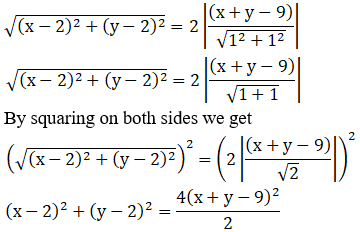[We know that (a – b)2 = a2 + b2 + 2ab &(a + b + c)2 = a2 + b2 + c2 + 2ab + 2bc + 2ac]

x2 + 4 – 4x + y2 + 4 – 4y = 2{x2 + y2 + 81 + 2xy – 18y – 18x}

x2 – 4x + y2 + 8 – 4y = 2x2 + 2y2 + 162 + 4xy – 36y – 36x

x2 – 4x + y2 + 8 – 4y – 2x2 – 2y2 – 162 – 4xy + 36y + 36x = 0

– x2 – y2 + 32x + 32y + 4xy – 154 = 0

x2 + 4xy + y2 – 32x – 32y + 154 = 0

∴The equation of hyperbola isx2 + 4xy + y2 – 32x – 32y + 154 = 0

3. Find the eccentricity, coordinates of the foci, equations of directrices and length of the latus-rectum of the hyperbola.
(i) 9x2 – 16y2 = 144

(ii) 16x2 – 9y2 = -144

(iii) 4x2 – 3y2 = 36

(iv) 3x2 – y2 = 4

(v) 2x2 – 3y2 = 5

Solution:

(i) 9x2 – 16y2 = 144

Given:

The equation => 9x2 – 16y2 = 144

The equation can be expressed as: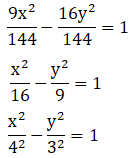The obtained equation is of the form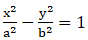Where, a2 = 16, b2 = 9 i.e., a = 4 and b = 3

Eccentricity is given by: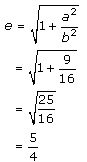Foci: The coordinates of the foci are (0, ±be)

(0, ±be) = (0, ±4(5/4))

= (0, ±5)

The equation of directrices is given as: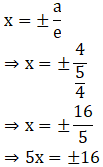5x ∓ 16 = 0

The length of latus-rectum is given as:

2b2/a

= 2(9)/4

= 9/2

(ii) 16x2 – 9y2 = -144

Given:

The equation => 16x2 – 9y2 = -144

The equation can be expressed as: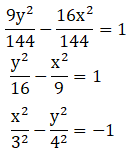The obtained equation is of the formWhere, a2 = 9, b2 = 16 i.e., a = 3 and b = 4

Eccentricity is given by: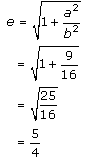Foci: The coordinates of the foci are (0, ±be)

(0, ±be) = (0, ±4(5/4))

= (0, ±5)

The equation of directrices is given as: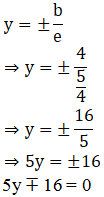The length of latus-rectum is given as:

2a2/b

= 2(9)/4

= 9/2

(iii) 4x2 – 3y2 = 36

Given:

The equation => 4x2 – 3y2 = 36

The equation can be expressed as: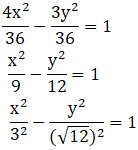The obtained equation is of the formWhere, a2 = 9, b2 = 12 i.e., a = 3 and b = √12

Eccentricity is given by: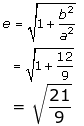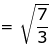Foci: The coordinates of the foci are (±ae, 0)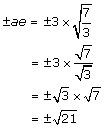(±ae, 0) = (±√21, 0)

The equation of directrices is given as: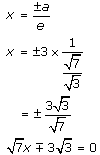The length of latus-rectum is given as:

2b2/a

= 2(12)/3

= 24/3

= 8

(iv) 3x2 – y2 = 4

Given:

The equation => 3x2 – y2 = 4

The equation can be expressed as: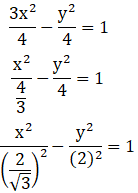The obtained equation is of the formWhere, a = 2/√3 and b = 2

Eccentricity is given by: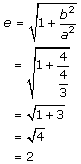Foci: The coordinates of the foci are (±ae, 0)

(±ae, 0) = ±(2/√3)(2) = ±4/√3

(±ae, 0) = (±4/√3, 0)

The equation of directrices is given as: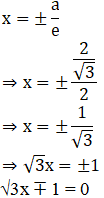The length of latus-rectum is given as:

2b2/a

= 2(4)/[2/√3]

= 4√3

(v) 2x2 – 3y2 = 5

Given:

The equation => 2x2 – 3y2 = 5

The equation can be expressed as: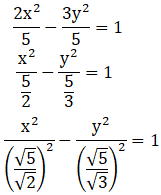The obtained equation is of the formWhere, a = √5/√2 and b = √5/√3

Eccentricity is given by: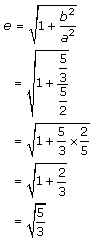Foci: The coordinates of the foci are (±ae, 0)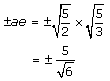(±ae, 0) = (±5/√6, 0)

The equation of directrices is given as: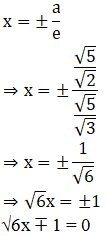The length of latus-rectum is given as:

2b2/a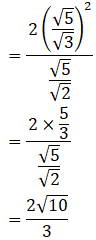4. Find the axes, eccentricity, latus-rectum and the coordinates of the foci of the hyperbola 25x2 – 36y2 = 225.

Solution:

Given:

The equation=> 25x2 – 36y2 = 225

The equation can be expressed as: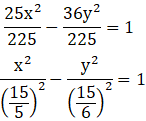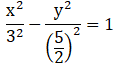The obtained equation is of the formWhere, a = 3 and b = 5/2

Eccentricity is given by: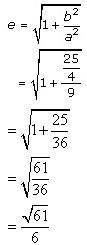Foci: The coordinates of the foci are (±ae, 0)

(±ae, 0) = ±3 (√61/6) = ± √61/2

(±ae, 0) = (± √61/2, 0)

The equation of directrices is given as: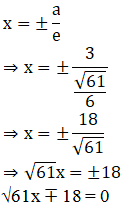The length of latus-rectum is given as:

2b2/a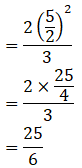∴ Transverse axis = 6, conjugate axis = 5, e = √61/6, LR = 25/6, foci = (± √61/2, 0)

5. Find the centre, eccentricity, foci and directions of the hyperbola
(i) 16x2 – 9y2 + 32x + 36y – 164 = 0

(ii) x2 – y2 + 4x = 0

(iii) x2 – 3y2 – 2x = 8

Solution:

(i) 16x2 – 9y2 + 32x + 36y – 164 = 0

Given:

The equation => 16x2 – 9y2 + 32x + 36y – 164 = 0

Let us find the centre, eccentricity, foci and directions of the hyperbola

By using the given equation

16x2 – 9y2 + 32x + 36y – 164 = 0

16x2 + 32x + 16 – 9y2 + 36y – 36 – 16 + 36 – 164 = 0

16(x2 + 2x + 1) – 9(y2 – 4y + 4) – 16 + 36 – 164 = 0

16(x2 + 2x + 1) – 9(y2 – 4y + 4) – 144 = 0

16(x + 1)2 – 9(y – 2)2 = 144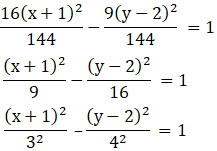Here, center of the hyperbola is (-1, 2)

So, let x + 1 = X and y – 2 = Y

The obtained equation is of the formWhere, a = 3 and b = 4

Eccentricity is given by: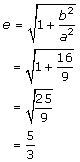Foci: The coordinates of the foci are (±ae, 0)

X = ±5 and Y = 0

x + 1 = ±5 and y – 2 = 0

x = ±5 – 1 and y = 2

x = 4, -6 and y = 2

So, Foci: (4, 2) (-6, 2)

Equation of directrix are: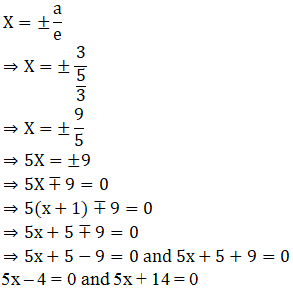∴ The center is (-1, 2), eccentricity (e) = 5/3, Foci = (4, 2) (-6, 2), Equation of directrix = 5x – 4 = 0 and 5x + 14 = 0

(ii) x2 – y2 + 4x = 0

Given:

The equation => x2 – y2 + 4x = 0

Let us find the centre, eccentricity, foci and directions of the hyperbola

By using the given equation

x2 – y2 + 4x = 0

x2 + 4x + 4 – y2 – 4 = 0

(x + 2)2 – y2 = 4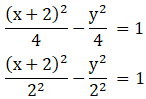Here, center of the hyperbola is (2, 0)

So, let x – 2 = X

The obtained equation is of the formWhere, a = 2 and b = 2

Eccentricity is given by:Foci: The coordinates of the foci are (±ae, 0)

X = ± 2√2 and Y = 0

X + 2 = ± 2√2 and Y = 0

X= ± 2√2 – 2 and Y = 0

So, Foci = (± 2√2 – 2, 0)

Equation of directrix are: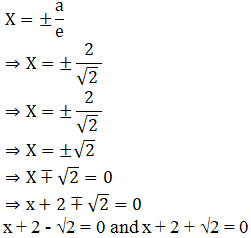∴ The center is (-2, 0), eccentricity (e) = √2, Foci = (-2± 2√2, 0), Equation of directrix =

x + 2 = ±√2

(iii) x2 – 3y2 – 2x = 8

Given:

The equation => x2 – 3y2 – 2x = 8

Let us find the centre, eccentricity, foci and directions of the hyperbola

By using the given equation

x2 – 3y2 – 2x = 8

x2 – 2x + 1 – 3y2 – 1 = 8

(x – 1)2 – 3y2 = 9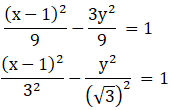Here, center of the hyperbola is (1, 0)

So, let x – 1 = X

The obtained equation is of the formWhere, a = 3 and b = √3

Eccentricity is given by: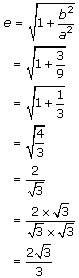Foci: The coordinates of the foci are (±ae, 0)

X = ± 2√3 and Y = 0

X – 1 = ± 2√3 and Y = 0

X= ± 2√3 + 1 and Y = 0

So, Foci = (1 ± 2√3, 0)

Equation of directrix are: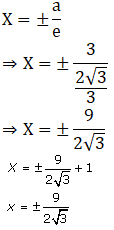∴ The center is (1, 0), eccentricity (e) = 2√3/3, Foci = (1 ± 2√3, 0), Equation of directrix =

X = 1±9/2√3

6. Find the equation of the hyperbola, referred to its principal axes as axes of coordinates, in the following cases:
(i) the distance between the foci = 16 and eccentricity = √2

(ii) conjugate axis is 5 and the distance between foci = 13

(iii) conjugate axis is 7 and passes through the point (3, -2)

Solution:

(i) the distance between the foci = 16 and eccentricity = √2

Given:

Distance between the foci = 16

Eccentricity = √2

Let us compare with the equation of the formDistance between the foci is 2ae and b2 = a2(e2 – 1)

So,

2ae = 16

ae = 16/2

a√2 = 8

a = 8/√2

a2 = 64/2

= 32

We know that, b2 = a2(e2 – 1)

So, b2 = 32 [(√2)2 – 1]

= 32 (2 – 1)

= 32

The Equation of hyperbola is given as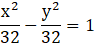x2 – y2 = 32

∴ The Equation of hyperbola is x2 – y2 = 32

(ii) conjugate axis is 5 and the distance between foci = 13

Given:

Conjugate axis = 5

Distance between foci = 13

Let us compare with the equation of the formDistance between the foci is 2ae and b2 = a2(e2 – 1)

Length of conjugate axis is 2b

So,

2b = 5

b = 5/2

b2 = 25/4

We know that, 2ae = 13

ae = 13/2

a2e2 = 169/4

b2 = a2(e2 – 1)

b2 = a2e2 – a2

25/4 = 169/4 – a2

a2 = 169/4 – 25/4

= 144/4

= 36

The Equation of hyperbola is given as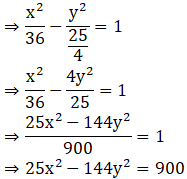∴ The Equation of hyperbola is 25x2 – 144y2 = 900

(iii) conjugate axis is 7 and passes through the point (3, -2)

Given:

Conjugate axis = 7

Passes through the point (3, -2)

Conjugate axis is 2b

So,

2b = 7

b = 7/2

b2 = 49/4

The Equation of hyperbola is given asSince it passes through points (3, -2)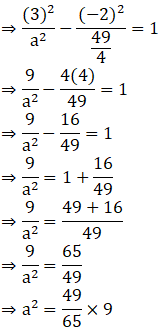a2 = 441/65

The equation of hyperbola is given as: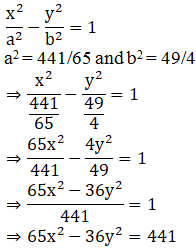∴ The Equation of hyperbola is 65x2 – 36y2 = 441

## Frequently Asked Questions on RD Sharma Solutions for Class 11 Maths Chapter 27

### What are the benefits of referring to the RD Sharma Solutions for Class 11 Maths Chapter 27?

Every student has to take up exams as they are the basis of assessing how knowledgeable and informational a student is. The RD Sharma Solutions for Class 11 Maths Chapter 27 provide precise and detailed answers to the textbook questions. All the concepts are explained in simple steps to aid students to ace their annual exam. Students can also improve their problem solving abilities and time management skills by regularly using these resources while preparing for exams.

### Do the RD Sharma Solutions for Class 11 Maths Chapter 27 follow the CBSE guidelines?

The RD Sharma Solutions for Class 11 Maths Chapter 27, prepared by the expert tutors at BYJU’S strictly adheres to the CBSE guidelines. Each and every solution is solved with utmost care with the aim of helping students to perform well in the board exam. By using the RD Sharma Solutions, students will be able to grasp the difficult concepts in the chapter and clarify the doubts instantly. The interactive explanation provided for each answer enables confidence among students, which are important to score more marks in the Class 11 exam.

### Why should students opt for BYJU’S RD Sharma Solutions for Class 11 Maths Chapter 27 as reference material?

Students should opt for the BYJU’S RD Sharma Solutions for Class 11 Maths Chapter 27 as it’s handy and easy to understand. All the solutions are prepared by subject experts at BYJU’S using simple language for the ease of learning. Moreover, the solutions can be viewed online and downloaded in PDF for offline use by the students for free and without any time constraints. Neat explanations using steps in the solutions help students learn answer presentation skills vital for exams.